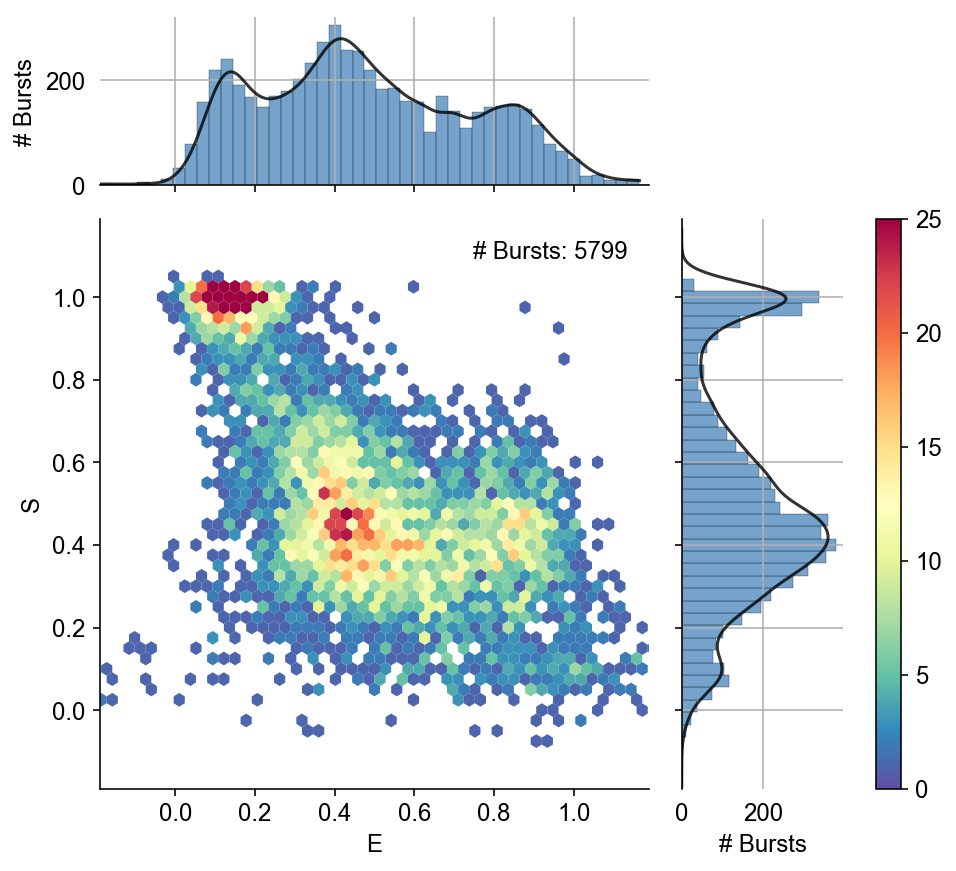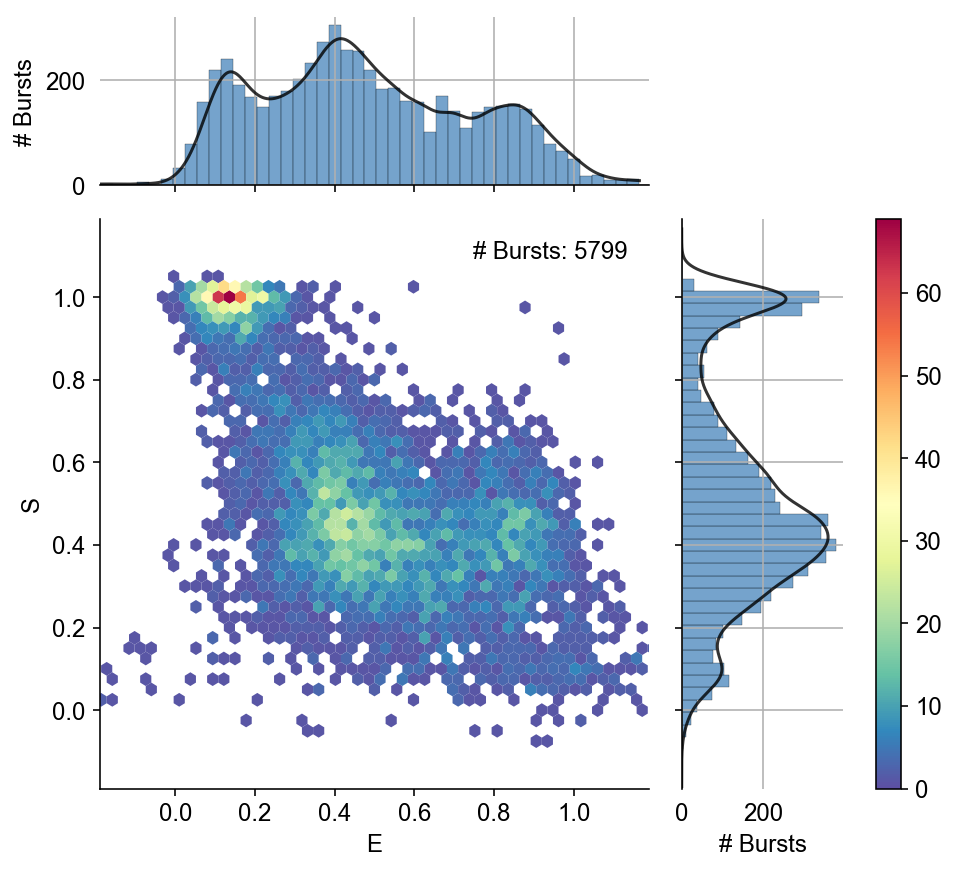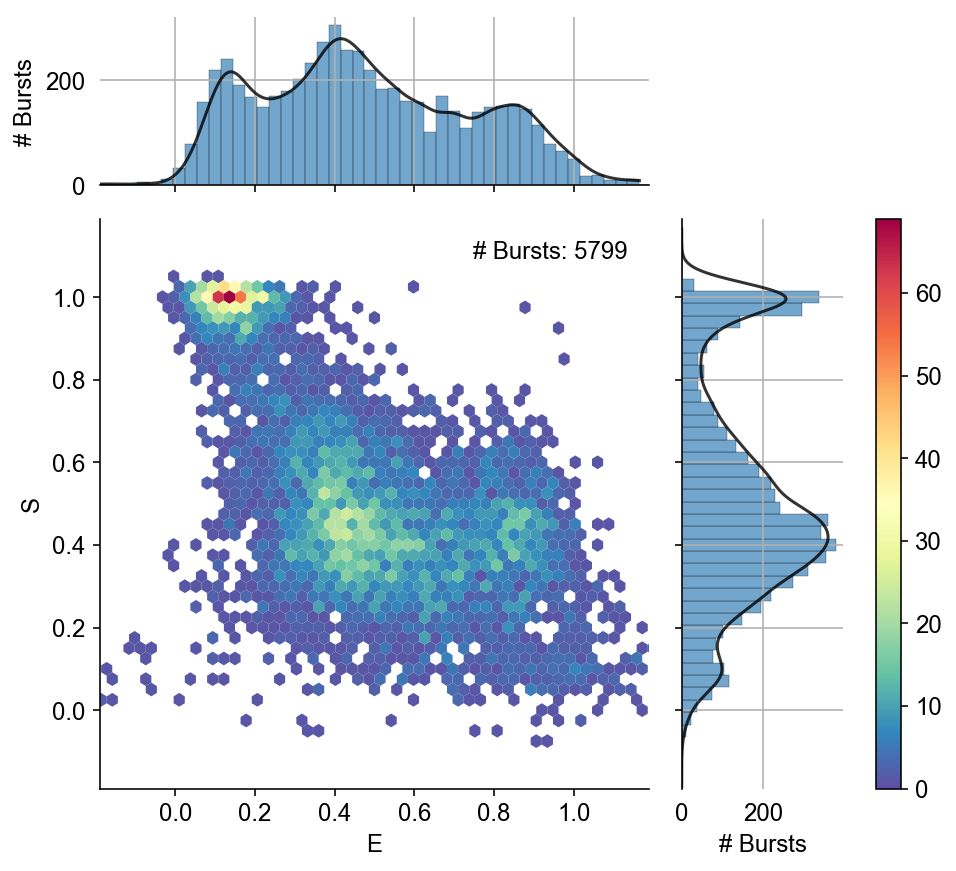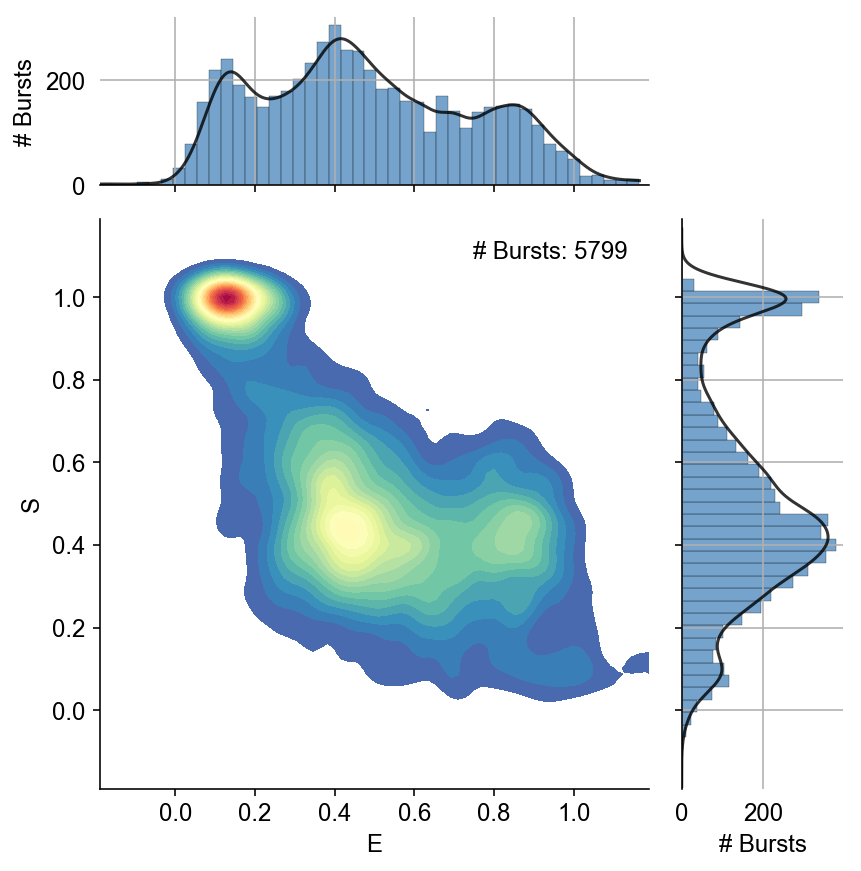# Example - Customize the μs-ALEX histogram¶

This notebook is part of smFRET burst analysis software FRETBursts.

In this notebook shows how to plot different styles of μs-ALEX histograms and $E$ and $S$ marginal distributions. For a complete tutorial on burst analysis see FRETBursts - us-ALEX smFRET burst analysis.

In :
from fretbursts import *

 - Optimized (cython) burst search loaded.
- Optimized (cython) photon counting loaded.
--------------------------------------------------------------
You are running FRETBursts (version 0.6.4).

If you use this software please cite the following paper:

FRETBursts: An Open Source Toolkit for Analysis of Freely-Diffusing Single-Molecule FRET
Ingargiola et al. (2016). http://dx.doi.org/10.1371/journal.pone.0160716

--------------------------------------------------------------

In :
sns = init_notebook(apionly=True)
print('seaborn version: ', sns.__version__)

seaborn version:  0.8.0

In :
# Tweak here matplotlib style
import matplotlib as mpl
mpl.rcParams['font.sans-serif'].insert(0, 'Arial')
mpl.rcParams['font.size'] = 12
%config InlineBackend.figure_format = 'retina'


# Get and process data¶

In :
url = 'http://files.figshare.com/2182601/0023uLRpitc_NTP_20dT_0.5GndCl.hdf5'
full_fname = "./data/0023uLRpitc_NTP_20dT_0.5GndCl.hdf5"

d.calc_bg(bg.exp_fit, time_s=1000, tail_min_us=(800, 4000, 1500, 1000, 3000))
d.burst_search(L=10, m=10, F=6)

URL:  http://files.figshare.com/2182601/0023uLRpitc_NTP_20dT_0.5GndCl.hdf5
File: 0023uLRpitc_NTP_20dT_0.5GndCl.hdf5

# Total photons (after ALEX selection):     2,259,522
#  D  photons in D+A excitation periods:      721,537
#  A  photons in D+A excitation periods:    1,537,985
# D+A photons in  D  excitation period:     1,434,842
# D+A photons in  A  excitation period:       824,680

- Calculating BG rates ... [DONE]
- Performing burst search (verbose=False) ...[DONE]
- Calculating burst periods ...[DONE]
- Counting D and A ph and calculating FRET ...
- Applying background correction.
[DONE Counting D/A]


# ALEX joint plot¶

The alex_jointplot function allows plotting an ALEX histogram with marginals. This is how it looks by default:

In :
alex_jointplot(ds)

Out:
<seaborn.axisgrid.JointGrid at 0x11da77dd8>The inner plot in an hexbin plot, basically a 2D histogram with hexagonal bins. This kind of histograms resembles a scatter plot when sample size is small, and is immune from grid artifacts typical of rectangular grids. For more info for hexbin see this document.

The marginal plots are histograms with an overlay KDE plot. The same FRETBursts function that plots standalone E and S histograms is used here to plot the marginals in the joint plot.

Below I show how to customize appearance and type of this plot.

## Changing colors¶

By default the colormap range is computed on the range S=[0.2, 0.8], so that the FRET populations (S ~ 0.5) have more contrast.

To normalize the colormap to the whole data use the vmax argument:

In :
alex_jointplot(ds, vmax_fret=False)

Out:
<seaborn.axisgrid.JointGrid at 0x11e804860>In :
alex_jointplot(ds, vmax_fret=False, marginal_color=8)

Out:
<seaborn.axisgrid.JointGrid at 0x1205ad208>In :
alex_jointplot(ds, vmax_fret=False, marginal_color=7)

Out:
<seaborn.axisgrid.JointGrid at 0x120294358>In :
alex_jointplot(ds, kind='kde')

Out:
<seaborn.axisgrid.JointGrid at 0x121217f28>Or you can manually choose the max value mapped by the colormap (vmax):

In :
alex_jointplot(ds, vmax=40)

Out:
<seaborn.axisgrid.JointGrid at 0x121cd0908>